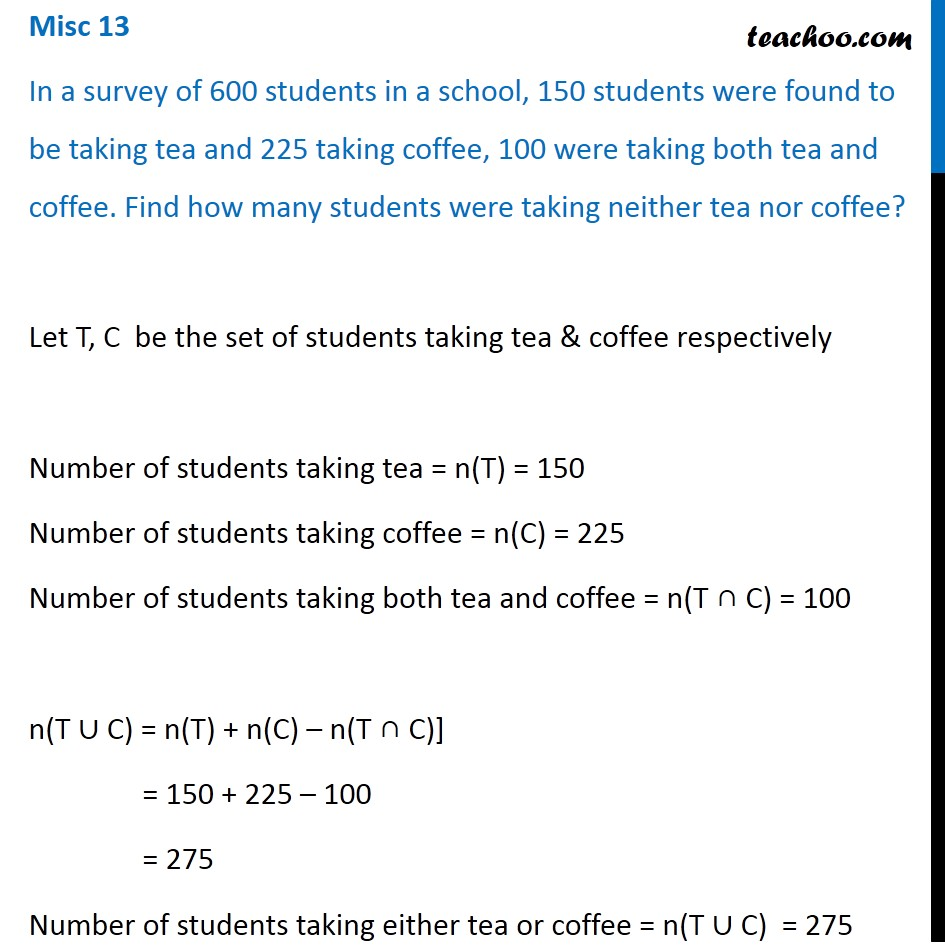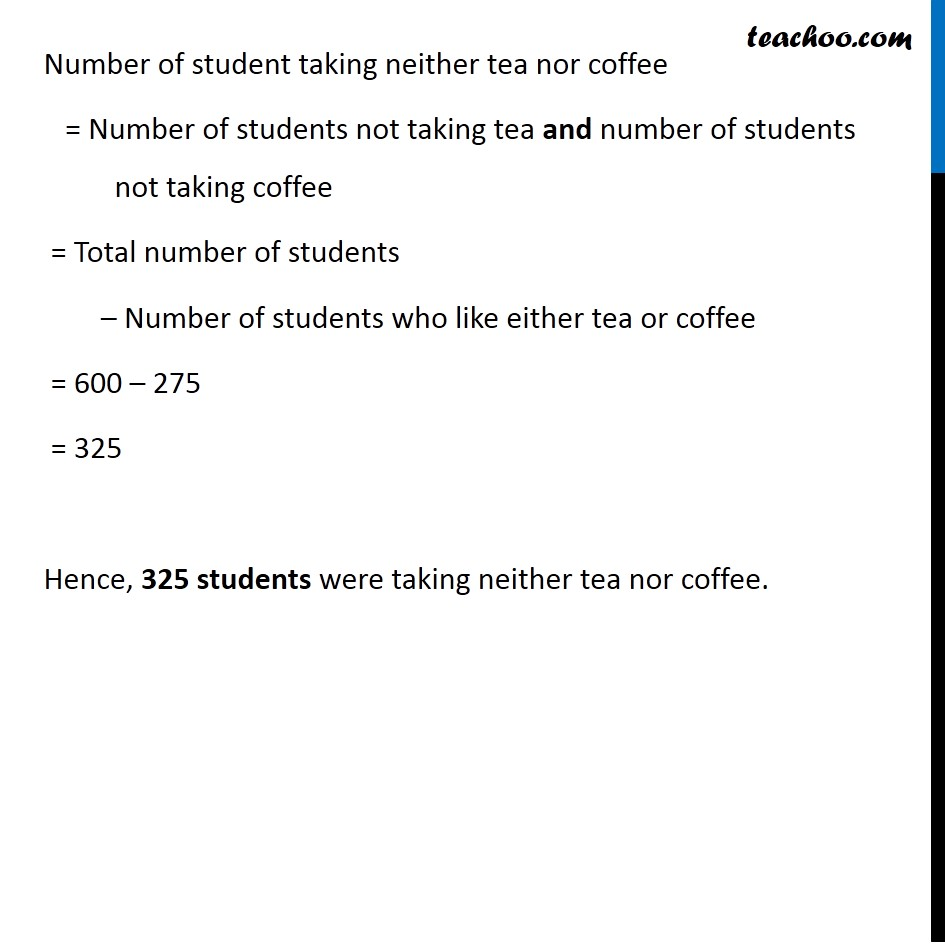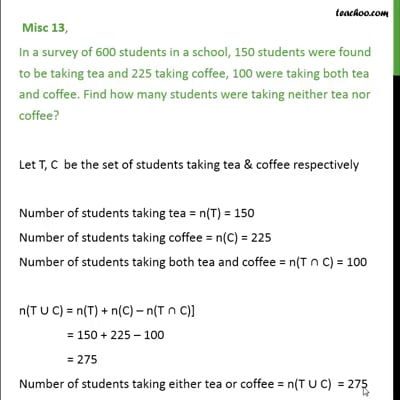Miscellaneous

Chapter 1 Class 11 Sets
Serial order wiseThis video is only available for Teachoo black users

Maths Crash Course - Live lectures + all videos + Real time Doubt solving!

### Transcript

Misc 13 In a survey of 600 students in a school, 150 students were found to be taking tea and 225 taking coffee, 100 were taking both tea and coffee. Find how many students were taking neither tea nor coffee? Let T, C be the set of students taking tea & coffee respectively Number of students taking tea = n(T) = 150 Number of students taking coffee = n(C) = 225 Number of students taking both tea and coffee = n(T ∩ C) = 100 n(T ∪ C) = n(T) + n(C) – n(T ∩ C)] = 150 + 225 – 100 = 275 Number of students taking either tea or coffee = n(T ∪ C) = 275 Number of student taking neither tea nor coffee = Number of students not taking tea and number of students not taking coffee = Total number of students – Number of students who like either tea or coffee = 600 – 275 = 325 Hence, 325 students were taking neither tea nor coffee.